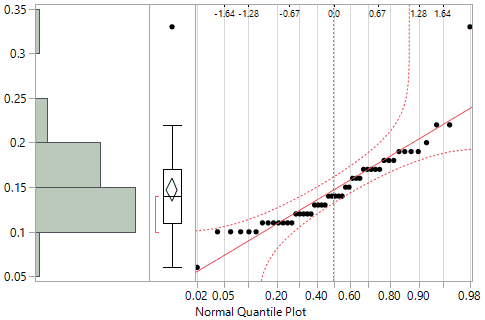Publication date: 04/12/2021

## Normal Quantile Plot

Use the Normal Quantile Plot option to visualize the extent to which the variable is normally distributed. If a variable is normally distributed, the normal quantile plot approximates a diagonal straight line. This type of plot is also called a quantile-quantile plot, or Q-Q plot.

The normal quantile plot also shows Lilliefors confidence bounds (Conover 1980) and probability and normal quantile scales.

Figure 3.7 Normal Quantile PlotNote the following information:

The vertical axis shows the column values.

The upper horizontal axis shows the normal quantile scale.

The lower horizontal axis shows the empirical cumulative probability for each value.

The dashed red line shows the Lilliefors confidence bounds.

For statistical details, see Normal Quantile Plot.

Want more information? Have questions? Get answers in the JMP User Community (community.jmp.com).